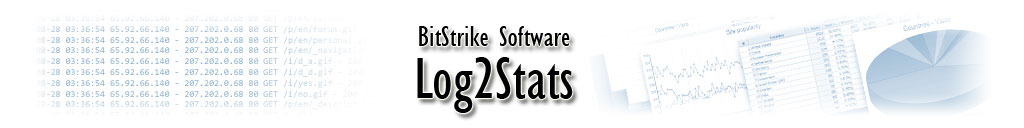## Log2Stats Documentation

#### Contents

##### Custom log file format

This section is about how to tell Log2Stats the format of your logfile. Most people don't need to do this because Log2Stats can detect the format automatically - try it first and see! But if it can't, and you need to specify the log format explicitly, here is how to do it.

###### Log Formats that Log2Stats can detect automatically

Here is the list of log file formats with their corresponding format strings, which can be detected by Log2Stats automatically. This list does not include W3C Extended log file format because it does not require format string.
In some rare cases Log2Stats will not detect log file format properly. This situation occurs when the log file has numeric month representation and day of the month is not greater than 12. For example, when you import log for 7th of January,2010 the date can be in two formats - 07/01/2010 or 01/07/2010, so Log2Stats can not figure out is it January, 7th or July, 1st. If you feel that Log2Stats faces this problem, then you should swap '%d' and '%m' in custom format string.

###### Common
```%S %j %u [%d/%M/%Y:%h:%n:%o%w%j] "%e%w%r%wHTTP%j" %c %b
%S %j %u [%d/%M/%Y:%h:%n:%o%w%j] "%e%w%r" %c %b
%S %j %u [%d/%M/%Y:%h:%n:%o%w%j] "%r" %c %b```
###### Microsoft Common
```%S %j %u [%d/%M/%Y:%h:%n:%o%w%j] "%e%w%r%w"HTTP%j" %c %b
%S %j %u [%d/%M/%Y:%h:%n:%o%w%j] "%e%w%r" %c %b
%S %j %u [%d/%M/%Y:%h:%n:%o%w%j] "%r" %c %b```
###### Combined (3-letter month name)
```%S %j %u [%d/%M/%Y:%h:%n:%o%w%j] "%e%w%r%wHTTP%j" %c %b "%f" "%B"
%S %j %u [%d/%M/%Y:%h:%n:%o%w%j] "%e%w%r" %c %b "%f" "%B"
%S %j %u [%d/%M/%Y:%h:%n:%o%w%j] "%r" %c %b \"%f" "%B"```
###### Combined (International dates)
```%S %j %u [%d/%m/%Y:%h:%n:%o%w%j] "%e%w%r%wHTTP%j" %c %b "%f" "%B"
%S %j %u [%d/%m/%Y:%h:%n:%o%w%j] "%e%w%r" %c %b "%f" "%B"
%S %j %u [%d/%m/%Y:%h:%n:%o%w%j] "%r" %c %b "%f" "%B"```
###### Combined (North American dates)
```%S %j %u [%m/%d/%Y:%h:%n:%o%w%j] "%e%w%r%wHTTP%j" %c %b "%f" "%B"
%S %j %u [%m/%d/%Y:%h:%n:%o%w%j] "%e%w%r" %c %b "%f" "%B"
%S %j %u [%m/%d/%Y:%h:%n:%o%w%j] "%r" %c %b "%f" "%B"```
###### Microsoft log (North American dates)
```%S, %u, %m/%d/%y, %h:%n:%o, W3SVC%j, %j, %v, %T, %j, %b, %c, %j, %e, %r, %q,
%S, %u, %m/%d/%Y, %h:%n:%o, W3SVC%j, %j, %v, %T, %j, %b, %c, %j, %e, %r, %q,```
###### Microsoft log (International dates)
```%S, %u, %d/%m/%y, %h:%n:%o, W3SVC%j, %j, %v, %T, %j, %b, %c, %j, %e, %r, %q,
%S, %u, %d/%m/%Y, %h:%n:%o, W3SVC%j, %j, %v, %T, %j, %b, %c, %j, %e, %r, %q,```
###### WebSite log (North American dates)
```%m/%d/%y %h:%n:%o\t%S\t%v\t%j\t%u\t%e\t%r\t%f\t%j\t%B\t%c\t%b\t%T
%d/%m/%y %h:%n:%o\t%S\t%v\t%j\t%u\t%e\t%r\t%f\t%j\t%B\t%c\t%b\t%T```
###### Format string specification

If your logfile is not in one of the recognised formats, you can tell Log2Stats about your format using a log format string. You will need this if your logfile has lines which are not in one of the standard formats.

The format string consists of a template for the logfile line, with the various fields and special characters replaced by codes as follows. Please note that these codes are case sensitive -- for example, %b is completely different from %B!

Format codeDescription
%Shost (the client hostname, or address of the computer making the request)
%snumerical IP address of client (if recorded in a separate field; used when %S is empty)
%Lserver name (for load balancing support)
%rfile requested
%REquals to "%e%w%r%wHTTP%j"
%qquery string (part of filename after '?', if recorded in a separate field)
%Bbrowser
%Abrowser with +'s instead of spaces
%freferrer
%emethod
%uuser (tip: a cookie can usefully be defined as %u too)
%vvirtual host (the server hostname, also called the virtual domain)
%dday of the month
%mmonth in digits
%Mmonth, three letter English abbreviation
%yyear, last two digits
%Yyear, four digits
%Zyear, two or four digits (less efficient)
%hhour of the day
%nminute of the hour
%osecond of the minute
%aa or A for am, or p or P for pm, if %h is in the 12-hour clock. (So to match "am" you need %am and to match "AM" you need %aM)
%U"Unix time" (seconds since beginning of 1970, GMT). If it includes decimals, use %U.%j
%bnumber of bytes transferred
%inumber of bytes received
%tprocessing time in seconds
%Tprocessing time in milliseconds
%cHTTP status code
%jjunk: ignore this field (field can be empty too)
%wwhite space: spaces or tabs
%Woptional white space
%%% sign
\ttab stop
\\single backslash